# How to: Sum, Mean, Variance, Covariance in Linear Algebra

If the title looks puzzling let me say that I believe that learning Python (or R) will take you to Machine Learning, but learning Linear Algebra will take you everywhere.

So going from using sw libraries to creating your stuff means moving with agility from numbers to vectors (and matrices) and often translating “Summations” expressions into equivalent one shot Vector/Matrix operations.

Unfortunately moving between these two worlds has some traps for beginners that might be good to refresh as they come up often at later stage in more complex situations.Photo by Tiaan van Zyl on Unsplash

When we begin learning Linear Algebra (LA) the first chapters are often about elementary concepts about vectors and vector operations. Most of the times those of us who have a tech/scientific background at least, skip ahead because the first concepts are considered trivial. But if you well check some exercises and demonstrations they are not so trivial unless you already have confidence in translating math in algebra and vice versa.

To warm up assume for example you have a simple vector of n=5 elements X=[5,4,1,3,7] and you want the sum of its numbers. We can represent in mathematical terms with the sum symbol like this: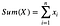(1)

If we want to represent the same thing in Linear Algebra with a data vector we learn we could use the dot product definition, but in that case what’s the second vector we should use ?

In this simple case could be good defining a second vector J of ‘1’s of the same dimension of the x vector. [In Python this could be: J=np.ones((1,5),int)]

So if we define for example J=[1,1,1,1,1] then X∙J gives us the SUM of X as (5・1)+(4・1)+(1・1)+(3・1)+(7・1) and this is something good to start with.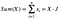(2) The Sum as Math and Algebra operations

2. Mean of numbers in a vector

From the above you can immediately determine the mean of a data vector with this: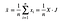(3) The mean of numbers in a vector in Math and Algebra

So far so good we defined sum and mean.

3. Centering data around the mean

At this point we have the elements to perform an operation that yes is simple but quite often happens in ML: the centering of a data vector around its mean. We can avoid alltogether the sum symbol with the first and as a proof the second: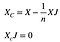Xc is the vector with data centered around X mean. As a proof the dot product should be zero

4. Variance for the numbers in a vector

When we look at some other math formulas like the one below, which is basically the formula of variance (note that I left out the 1/n or the 1/n-1 factor which exists in the variance formula) and we think about how to do that in Linear Algebra terms things are getting more fuzzy because our first thought is to develop the square of the binomial (something like: a²+b²-2ab) but doubts start arising as we have the summation symbol, then we have the mean which is also something with a summation in itself… so we must be careful.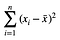(4)

If we look at the results of that in books they usually skip all the passages and present the result as in (5) and we believe, but then we ask: how did they go from (4) to (5) below ?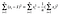(5)

The intuition of the square of the binomial is correct but with summations it’s better to be cautious. So in case you’re curious this is how to develop that:

Step 1: Develop the square of the binomial as usual leaving out the summation symbol: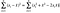(6)

Step 2: Only after you should distribute the summations symbols as this should avoid doubts like asking ourselves if the two things below are the same (they are not of course):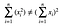Sometime squaring with sum symbol is a source of mistakes

So your development should be like this: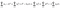(7) result after the distribution of the sum symbol

The first term is not reducible, while the second term could be source of confusion because it’s the sum of a value which has not the index i. So summing from i=1 to n, a constant value is … n times the value. For this second term we have in general the following :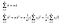(9)

Note: Just to convince you about the second line , suppose you have the vector X=[5,4,1,3,7]. This has mean of 4. Square is 16. The sum with i from 1 to 5 of 16 is equal to 16*5=80. As you can check in the last term the sum of the x elements is 20, square is 400, 400/5=80.

The third term of the (7) is also apparantely tricky but it’s not and it becomes: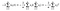(10)

Step 3: Putting all together we have this with the red part as the solution:

So we understood the development of the math formula, but how if we want to translate that same formula in a single line with Linear Algebra ?

To do that we can start from the result in red and dividing it in two pieces: the first line give us the sum of the product of X with itself. The second line is a consequence of what we wrote in line (2)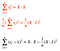Note the difference in the two formulas in red. Don’t think that X.X=X² or that (X.J)²=X²J² as they are not !!

Assuming for example X=[5,7,3], mean is 5, subtracting 5 from X and squaring: [0²+2²+(-2)²], so the sum is 8.

Same result with the formula in black becomes (25+49+9)–(225/3)=8 using the algebra formulas.

5. Simple Covariance

As an exercise, suppose you want to translate the formula for the simple Covariance between two variables X and Y. In this case you have: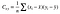Covariance formula for two random variables

From what we learned above we have the following steps:

Step 1: Explode the expression and Distribute the Sums

Step 2: Substitute and simplify where needed. Note that last term is a sum of a not indexed values so it becomes simply n times the product of the two means.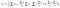note that in the last term the summation has been replaced by n. Note also that second, third, fourth term above are similars

Step 3: Collect and Simplify: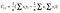Note the first term is a dot product between X and Y, the second term is the product of the sum of each vector

Step 4: Translate to Vectors

As you can see Linear Algebra looks easy but if you believe the approach you use for math works the same then be aware that this is not always true and mistakes are just round the corner.

I hope this can be of support in gradually improve your confidence with algebra elementary operations during your journey toward a better understanding of what’s behind the scenes of particular machine learning algorithms or statistics.

IT Senior Manager and Consultant. Data Warehouse and Business Intelligence expertise in design and build. Freelance.

## More from Andrea Grianti

IT Senior Manager and Consultant. Data Warehouse and Business Intelligence expertise in design and build. Freelance.